Lithology from Simultaneous Equations
Crossplot methods of the types discussed in other Chapters are actually solutions to three or four simultaneous equations. For example, the density neutron crossplot can be described by generalized forms of their response equations:

a1 * V1 + a2 * V2 + a3 * V3 = PHID
b1 * V1 + b2 * V2 + b3 * V3 = PHIN
1.0 * V1 + 1.0 * V2 + 1.0 * V3 = 1.00

Where:
a1, a2 and a3 are the density log porosity readings for rock components V1, V2 and V3
b1, b2 and b3 are the neutron log porosity readings for rock components V1, V2 and V3
V1, V2 and V3 are the rock volumes of the three components (fractional units)

Equation sets like this one, or larger sets, can be solved by Cramer's Rule. Some spreadsheet software can solve matrix algebra using Cramer's method.

The third equation is called the identity equation, and defines the fact that the three components add up to 1.00 (or 100%) of the formation seen by the logs. The first component could be porosity and the other two sand and shale or limestone and dolomite for example.

This concept can be extended to as many equations as there are independent log readings available on a well. For example a full suite of logs may consist of:

GR total,   uranium,   thorium,   potassium
density,   neutron,    sonic,     photoelectric effect
shallow resistivity,     medium resistivity,    deep resistivity
electromagnetic propagation

This, with the identity equation, gives a possibility of thirteen equations in thirteen unknowns. Add the   elemental capture yields from an ECS or the porosity breakdown from an NMR log and the list gets pretty unwieldy. It is unlikely that the three resistivity logs are truly independent equations, so they may not all assist. In addition, our knowledge of the rock response for all twelve logs and thirteen unknowns is not perfect, so it is possible that no solution or ridiculous solutions might result.

This eventuality can be reduced by taking all combinations of say, five or six equations of five or six unknowns from the more numerous possible unknowns, and checking the results of each solution for reasonableness. Reasonableness might be described by taking only solutions in which the volumes are all within the range of -0.05 to 1.05 (i.e. no material can be present in large negative quantities or be greater than the volume of the rock). This is a limited form of linear programming, which is another name for linear simultaneous equation with constraints.

Other constraints, or tests of reasonableness, may involve maximum limits on porosity or accessory minerals.

If more log types (known data) are available, than unknowns (rock volumes), as in the situation described above, then the case is called over-determined. More than one reasonable answer could be found in an over-determined case.

One artificial method for selecting the desired solution from the various possible ones is to design a computer program to select the rock types most likely to be present, from an ordered list supplied by the analyst.

Another method is to scan the rock types found in all reasonable solutions and pick a set from the rocks that occur most frequently. This set may itself lead to an unreasonable solution, so the the next most frequent solution is then chosen and checked until a reasonable solution is found.

If the number of knowns and unknowns are equal, then the case is exactly determined and only one solution is possible. The solution may still be unreasonable or merely wrong because the choice of possible minerals was poor.

If there are fewer knowns than unknowns, then the case is called underdetermined. It cannot be solved in the conventional matrix method, but two other approaches exist.

One is to provide sufficient constraints and other equalities, reasonable for the situation, to make the case determined or over-determined. This is called linear programming, and is akin to pulling yourself up by your own boot straps. The solution usually reflects your preconceived opinion, but can supply surprisingly accurate results in well controlled situations.

The second approach is to provide some kind of control equation which will solve a determined case defined by the control data. The program may switch from one determined case to another depending on the answers and the controlling equation.

In all the above cases, the neutron log response equation is non-linear for sandstone and dolomite rocks. Since the non-linearity is a function of porosity (usually one of the answers), a second iteration can usually improve results. This is done by calculating new neutron coefficients from appropriate equations and then re-solving the matrices. Other response equations may also be non-linear, such as the gamma ray or sonic logs.

Three simultaneous equations (including the unity equation) can be solved graphically on an X-Y crossplot, such as the density neutron or sonic density crossplots. Many examples have been shown in previous Chapters.

Porosity independent lithology indicators such as DENSma, DELTma, Mlith, Nlith, Alith, Klith, PEma, or Uma can be combined in pairs in three simultaneous equations, with the unity equation to solve for three unknowns. Because they are porosity independent terms, porosity cannot be one of the unknowns. These sets of equations are usually known as three mineral models, and can be solved by matrix algebra or by algebraic reduction as shown in the algorithms described in previous Chapters.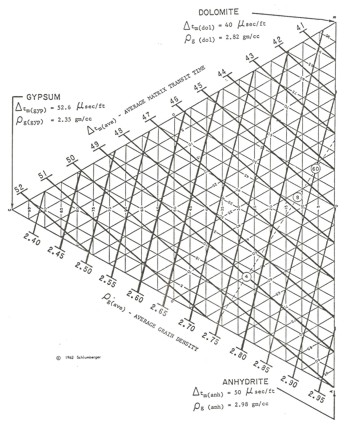Note that these solutions give three rock volumes summing to 100%. Unless porosity and shale are two of the “minerals”, each answer must be reduced by a fraction equal to (1 - PHIe - Vsh) as was done for two mineral models in previous Chapters.

Four simultaneous equations can be solved using triangular graph paper, such as that shown at the right.

The corners of the plot represent 100% of the three major rock components. An overlay of log value lines are drawn to linearly interpolate between corners so the log data can be plotted and lithology determined. Once the three rock volumes are found, porosity is calculated from an appropriate porosity log response equation.

Triangular graph paper to solve for lithology

In order to illustrate the variety of models which can be solved by simultaneous equations. Five examples are cited here.Example 1: Evaporite Model
The minerals sought are halite (W), sylvite (X), carnallite (Y), and insolubles or clay (Z). The only logs available on old wells are resistivity, sonic, neutron, and total gamma ray. The resistivity is not a helpful discriminator so it is not used. These evaporite beds contain potassium and ore grade is measured in units of potassium oxide (K2O). K20 is obtained from a gamma ray log, corrected for borehole size and mud weight, using a non-linear transform derived from core assay data. In middle aged wells, the density log is also helpful, and in modern wells the PE curve can be added. Further, the gamma ray response is linear on modern wells so the transform to K2O is not as critical.

The equations are:
1.00 = W + X + Y + Z
K20 = 0.63 * Z + 0.17 * Y + 0.05 * Z
PHIN = 0.65 * Y - 0.30 * Z
DTC = 67 * W + 74 * X + 78 * Y + 120 * Z (English units)

When solved by algebraic means, these become:
INSOLUBLES = Z = 0.0207 * DTC - 0.23 * K20 - 0.29 * PHIN - 1.3891
CARNALITE =    Y = 1.54 * PHIN - 0.46 * Z
SYLVITE =         X = 1.59 * K20 - 0.41 * PHIN + 0.04 * Z
HALITE =           W = 1.00 - X - Y - Z

To convert from mineral fraction to K2O equivalent (K2O equivalent is the way potash ores are rated), the final analysis follows:
K2O sylvite = 0.63 * X
K2O carnalite = 0.17 * Y
K2O total = K2O sylvite + K2O carnalite - 0.05 * Z

If these equations had been solved by matrix inversion, the solution equations would have appeared differently, but would have provided the same answer. In this example, the K20 log is derived from the GR separately because the relationship is non-linear.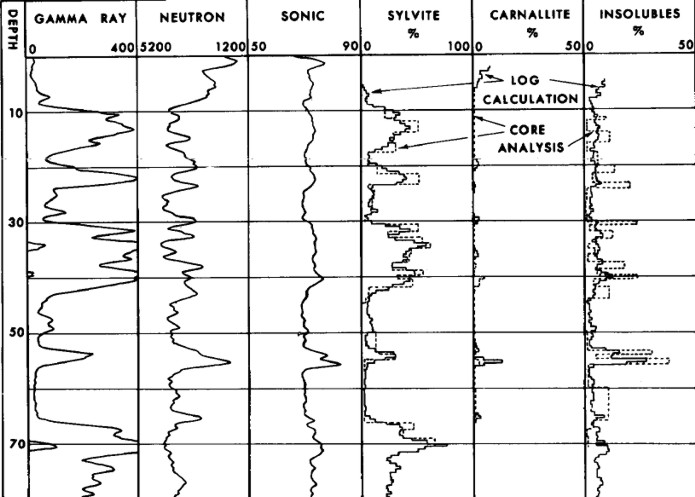Example log analysis showing excellent match to core data (circa 1964). Raw data is shown but note the scales are opposite polarity to normal.

If water (V) is added to the desired results (included in the salt or in the clay), the equations become:
1.00 = V + W + X + Y + Z

Where V = PHIN value in pure salt above the zone of interest.

The included water has zero gamma ray emission so the second equation remains unchanged:
K20total = 0.63 * X + 0.17 * Y - 0.05 * Z

The porosity is read directly by the neutron log, hence, the third equation becomes:
PHIN = 1.00 * V + 0.65 * Y + 0.30 * Z

The sonic equation becomes:
DTC = C + 67 * W + 74 * X + 78 * Y + 120 * Z

Where C = DTC in salt minus 67

Reduction of these equations results in:
Z = 0.0207 * (DTC - C) - 2.23 * K20 - 0.29 * (PHIN - V) - 1.3891
Y = 1.54 * (PHIN - V) - 0.64 * Z
X = 1.59 * K20 - 0.41 * (PHIN - V) - 0.04 * Z
W = 1.00 - X - Y - Z - V

Conversion to K20 equivalent remains the same as before.

These equations show the use of constraints (V and C) on the otherwise linear simultaneous equations. The first set of equations is exactly determined, and the second set are underdetermined until V and C are defined.

If the density equation were added, then the set would be exactly determined and the strategy of finding V and C in the pure salt bed would not be needed. This work was done in Saskatchewan before density logs were common, so the density equation was not used at that time.Example 2: Carbonate-Sand-Clay Model
This is an underdetermined case, with a control equation to allow the program to automatically switch to the correct set of four unknowns. Two or three mineral models with a trigger for a non-porous mineral such as coal or anhydrite, behave in a similar way.

DTC = 188 * PHIe + 43 * PHIsec + 43 * D + 47 * L + 56 * S + 51 * A + 100 * C1 + 150 * C2
DENS = 1.03*PHIe + 1.03*PHIsec + 2.86*D + 2.71*L + 2.65*S + 2.98*A + 2.65*C1+2.30*C2
PHIN = 1.00 * PHIe + 1.00 * PHIsec + W * D + L + X * S + Y * A + 0.28 * C1 + 0.48 * C2
1.0 = PHIe + PHIsec + D + L + S + A + C1 + C2
Z = PHIe + 150 * PHIsec + 15 * D + 6 * L + 80 * S + 500 * A + 60 * C1 + 150 * C2 (control equation)

Where:
DTC, DENS, PHIN = input data from sonic, density and neutron log readings
D, L, S, A, C1, C2 = percentages of dolomite, limestone, sandstone, anhydrite, and two types of shale
PHIe = primary porosity
PHIsec = secondary porosity (defined as porosity not seen by the sonic log)
W, X, Y = dolomite, sandstone and anhydrite coefficients to account for non linear neutron responseExample 3: Carbonate-Gypsum-Silica Model
This is also an underdetermined case with a constraint which defines how much silica to include in the final solution:
DTC = 185 * PHIe + 42 * D + 50 * A + 53 * G + 55 * S
DENS = 1.1 * PHIe + 2.84 * D + 2.98 * A + 2.35 * G + 2.65 * S
PHIN = 1.0 * PHIe + 0.02 * D + 0.0 * A + 0.49 * G - 0.04 * S
1.00 = 1.0 * PHIe + 1.0 * D + 1.0 * A + 1.0 * G + 1.0 * S
S = 0.0 + 0.0 + 0.0 + 0.0 + 1.0 * S

Where:
DTC, DENS, PHIN = the input values from sonic, density and neutron log respectively
PHIe = porosity
D, A, G, S = the computed fractions of bulk volume of dolomite, anhydrite, gypsum, and silica respectively

The inverse matrix solution for the above equations is:
PHIe = 0.0058 * DELT - 0.415 * DENS - 0.569 * PHIN + 0.945 - 0.189 * S
D = -0.030 * DELT - 6.59 * DENS - 8.28 * PHIN + 21.15 - 2.35 * S
A = 0.0352 * DELT + 5.889 * DENS + 5.315 * PHIN - 18.31 + 0.979 * S
G = -0.0106 * DELT + 1.116 * DENS + 3.542 * PHIN - 2.79 + 0.563 * S
S = 0.0 * DELT + 0.0 * DENS + 0.0 *PHIN + 0.0 + 1.0 * S

In this program, the equations are solved first with silica set to zero. If any of the other four components turns out to be negative, silica is added until that component goes to zero. This is considered the final solution to this underdetermined case.NUMERICAL EXAMPLE
Given log data of:
DTC = 51.4 usec/ft
DENS = 2.667 gm/cc
PHIN = 0.131

The results are:
PHIe = 0.063
D = 0.93
A = -0.10
G = 0.10
S = 0.00

Since A = -0.10 is impossible, S is raised until A is 0.0 giving:
PHIe = 0.040
D = 0.80
A = 0.00
G = 0.06
S = 0.10

Acceptance of the above solution is arbitrary because there are an infinite number of solutions for these three porosity log readings. For instance, if it were known that the silica fraction were 20 percent of the total, then the solution would be:
PHIe = 0.030
D = 0.46
A = 0.10
G = 0.21
S = 0.20Example 4: Carbonate Model
This example is underdetermined, but uses the frequency of occurrence of feasible (reasonable) solutions to switch the logic so that the most likely sub matrix that is exactly determined is computed.

The input parameters for the equations are shown below and all 70 combinations of 4x4 matrices taken from the 4x8 matrices are computed. Note that if 8 equations were available this set of unknowns would be determined, and only one solution would be possible.

All feasible solutions are tabulated, and the solution which contain the four rocks which occurred most often is circled. This was solution number 8 of the 70 possible solutions. Note that 28 solutions were feasible from the 70 that were possible.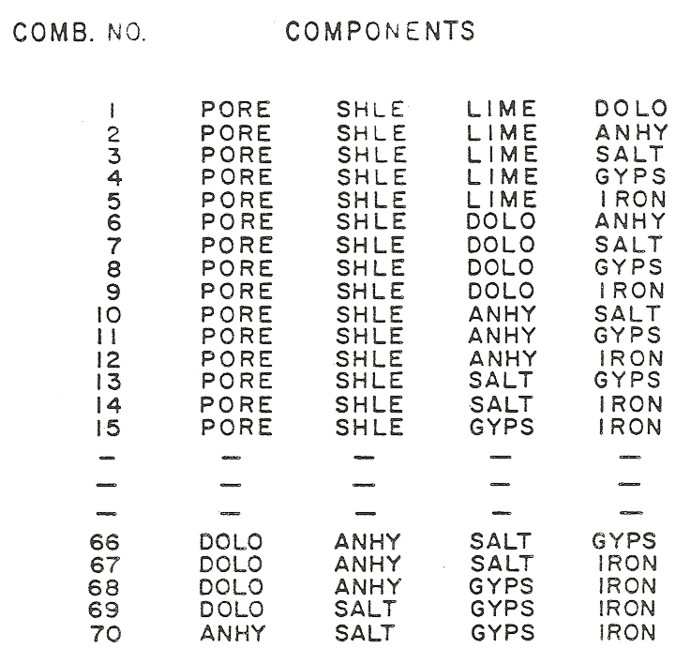Input parameters and 4 mineral model possibilities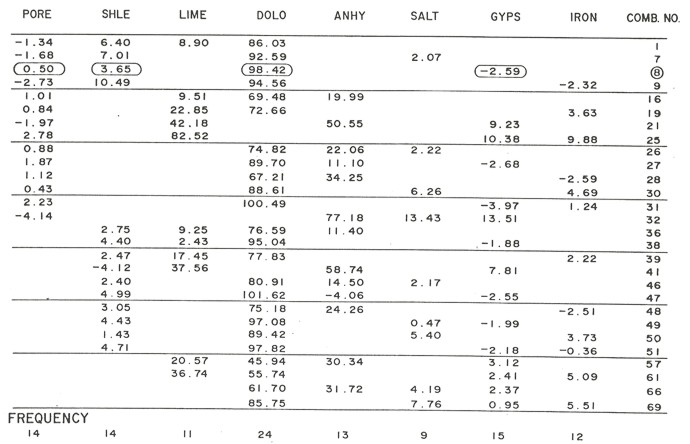Feasible solutions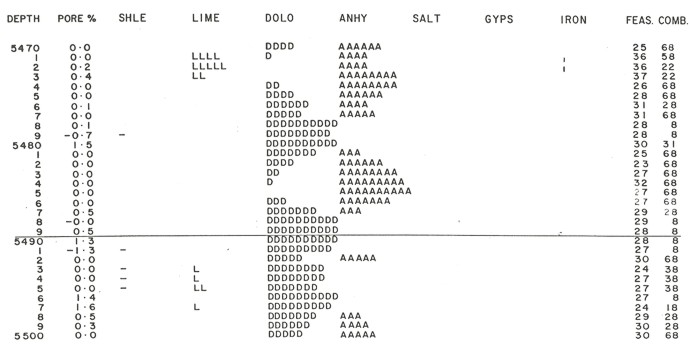Printer plot of lithology and porosityExample 5: Fluid Content Model
The usual log response equations are of the form:
LOG = PHIe * Sxo * Lw (water term)
+ PHIe * (1 - Sxo) * Lh (hydrocarbon term)
+ Vsh * Lsh (shale term)
+ (1 - Vsh - PHIe) * Sum (Vi * Li) (matrix term)

Where:
Lh = log reading in 100% hydrocarbon
Li = log reading in 100% of the ith component of matrix rock
Lsh = log reading in 100% shale
Lw = log reading in 100% water
PHIe = effective porosity (fractional)
Sxo = water saturation in invaded zone (fractional)
Vi = volume of ith component of matrix rock
Vsh = volume of shale (fractional)

This response equation will work for sonic travel time, density, or density porosity, neutron porosity, gamma ray (and the spectral GR curves - uranium, thorium, and potassium), resistivity (if Sxo is replaced by Sw for deep resistivity logs), the electromagnetic propagation log, the thermal decay time log, and the photoelectric effect (if PE * DENS is used).

The terms PHIe * Sxo and PHIe * (1 - Sxo) are the water volume and hydrocarbon volume in the invaded zone respectively.

The linear simultaneous equations can be used to determine these volumes. An example result is shown below, in which gas and water volumes were desired. This analysis used the same program as Example 4.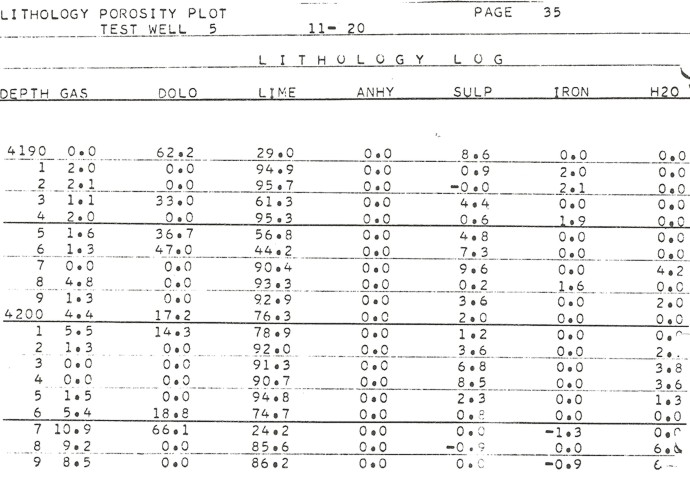Printer plot of lithology and fluid content

Gas plus water volumes add up to the effective porosity, and the ratio of water to effective porosity defines the invaded zone water saturation. The effective porosity could be used with a deep resistivity curve to obtain water saturation in the un-invaded zone, or the general purpose relation Sw = Sxo ^ 5 could be used.

This process is an expanded version of the bulk water concept. The water in the shale could be one of the desired components of the rock, and a dual water model would result.

Page Views ---- Since 01 Jan 2015
Copyright 2023 by Accessible Petrophysics Ltd.
CPH Logo, "CPH", "CPH Gold Member", "CPH Platinum Member", "Crain's Rules", "Meta/Log", "Computer-Ready-Math", "Petro/Fusion Scripts" are Trademarks of the Author